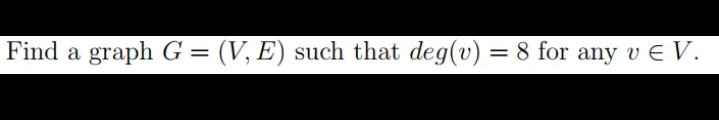Home / Expert Answers / Other Math / find-a-graph-g-v-e-such-that-deg-v-8-for-any-vv-pa143

# (Solved): Find a graph G=(V,E) such that deg(v)=8 for any vV. ...Find a graph such that for any .

We have an Answer from Expert

Given :

A graph G=(V,E) such that deg(v) = 8 for any v ? V .

If each vertex of the graph has the same degree k, the graph is called a k -regular graph

We have an Answer from Expert